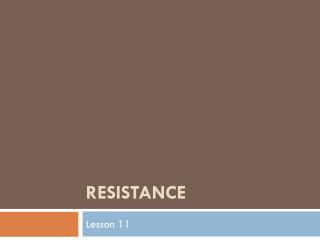DownloadDownload PresentationResistance

# Resistance

Télécharger la présentation## Resistance

- - - - - - - - - - - - - - - - - - - - - - - - - - - E N D - - - - - - - - - - - - - - - - - - - - - - - - - - -
##### Presentation Transcript

1. Resistance Lesson 11

2. Resistance • The degree to which a substance opposes the flow of electric current through it. • All substances resist electron flow to some extent.

3. Resistance • Conductors, such as metals, allow electrons to flow freely through them and have low resistance values. • Insulators resist electron flow greatly and have high resistance values.

4. Resistance • Resistance is measured in ohms (Ω)using an ohmmeter. • An ohmmeter is a device for measuring resistance. • Ohmmeters are connected in parallel

5. Resistance • When a substance resists the flow of electrons, it slows down the current and converts the electrical energy into other forms of energy. • The more resistance a substance has, the more energy gained by the substance is radiated to its surroundings as heat and/or light energy

6. Resistance in a Circuit • A resistor is any material that can slow current flow. • In a light bulb, the filament’s high resistance to the electron’s electrical energy causes it to heat up and produce light.

7. Resistors and Potential Difference • Resistors can be used to control current or potential difference in a circuit. • In a circuit, electrons have a higher potential difference as they enter a resistor compared to when they leave the resistor because they use up some energy in passing through the resistor.

8. Example: • Imagine electrons entering a resistor as being at the high end of a ramp, where they have a lot of potential energy. In this analogy, electrons leaving the resistor are at the bottom end of the ramp, where their potential energy has been converted to another form of energy

9. Types of Resistors • Resistors can be made with a number of techniques and materials • The two most common types are wire-wound and carbon-composition.

10. wire-wound resistor • A wire-wound resistor has a wire made of heat-resistant metal wrapped around an insulating core. The longer and thinner the wire, the higher the resistance. They are available with values from 0.1Ω up to 200 k Ω

11. Carbon-composition resistors • Carbon-composition resistors are made of carbon mixed with other materials. The carbon mixture is moulded into a cylinder with a wire at each end. By varying the size and composition of the cylinder, manufacturers produce resistances from 10 Ω to 20 M Ω. Moulded carbon resistors are cheaper to make than wire-wound resistors but less precise.

12. Resistance in a Wire –comparing water to electricity • Longer thinner pipes have more resistance to the flow of water than pipes with a larger diameter. The same idea applies to electricity. • The more resistance that you have in a circuit, the more it will decrease current at a given potential difference.

13. Larger, shorter wires provide less resistance for electrons to travel. • Temperature and material can also affect resistance.

14. Factors that Affect the Resistance of a Wire

15. Factors that Affect the Resistance of a Wire

16. Factors that Affect the Resistance of a Wire

17. Factors that Affect the Resistance of a Wire

18. Questions • Questions Page 566 #3,4,5,6,7